## Introduction

Individual earthquakes may be regarded as large scale ruptures involving a wide range of structural and compositional heterogeneities in the crust. However, the statistical properties of a population of earthquakes are often described by simple power-laws. Among them, two laws are ubiquitous and occupy a central position in statistical seismology: the Gutenberg-Richter (GR) law1 and the Modified Omori law (MOL)2,3. The GR law describes the earthquake magnitude-frequency distribution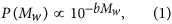where Mw is the moment magnitude and b a constant with a value around 1 along active fault zones. The MOL describes the aftershock occurrence rate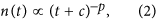where t is the elapsed time from the triggering event (the so-called mainshock), p a positive non-dimensional constant with a typical value of 1 and c a time constant. The parameters in these two laws are believed to bear some information on the physical state of the crust. Indeed, Schorlemmer et al. find that the b-value of the GR law is decreasing going from normal (extension) over strike-slip (shear) to thrust (compression) earthquakes4. Narteau et al. also find that the time constant c in the MOL has the same dependence on the faulting mechanism5. These two observations indicate that, under a simple assumption, b and c are decreasing functions of shear stress. Although the underlying mechanism needs further investigation, this may reflect a common time-dependent behaviour of fracturing in rocks during the propagation of earthquake ruptures and the nucleation of aftershocks.

Because the shear stress along an active fault is not directly measurable, a solution to address stress dependences in earthquake statistics is to analyse models that implement a restricted set of physical processes. Such models are indeed numerous, ranging from rock fracture experiments6,7,8,9 to computer simulations on cellular automata10,11. Among them, sheared granular media12,13,14,15,16,17,18,19 are simple representations of granular fault gouges (Fig. 1), which are commonly used in geophysics to analyse deformation of highly damaged rocks in fault zones20,21,22. Additionally, both the energy and the stress can be easily defined in these models.

Here we use the simplest form of structural complexity - a bimodal distribution of particle size - to elucidate its fundamental control on the emergent scaling laws. We then perform numerical simulations showing that, as for real seismicity, avalanches in sheared granular matter obeys the GR law and the MOL with b and c-values being decreasing functions of the shear stress. The details of our numerical model are described in the Methods section.

## Results

### Characterisation of avalanches

As observed in many previous works on amorphous particulate systems14,15,16,17,20,21,23,24,25,26,27,28,29,30, Fig. 2 shows that the temporal fluctuations of the energy becomes volatile if the shear rate is sufficiently low and the volume fraction is sufficiently high. Under such condition the kinetic energy is negligible in comparison with the elastic energy E(t) and therefore the elastic energy drop should approximate a transition from a local maximum to a local minimum in configurational energy. Then, an avalanche is defined as an abrupt drop of the elastic energy, E(t1) − E(t2), where t1 and t2 denote the beginning and the end of an event, respectively. This is illustrated in the inset of Fig. 2b.

Following the literature of earthquake studies31, we define the magnitude of avalanche as M ∫ log10[E(t1) − E(t2)] + m0, where m0 = 11 is chosen so that the magnitude of the smallest avalanches is approximately zero. Note that, with this definition of magnitude, the GR law reads P(M)  10−2/3bM, as the moment magnitude for earthquake31 is defined as Mw 2/3 log10[E(t1) − E(t2)] + const. Hereafter, we use β ∫ 2/3b instead of b. Other important quantities that characterise an avalanche are the initial stressand the stress drop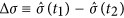. The definition of the shear stress and other technical remarks on avalanches are described in the Methods section.

### Magnitude-frequency distribution

First, we discuss the nature of the avalanche magnitude-frequency distribution with respect to the two control parameters, the shear rateand the volume fraction ϕ. Figure 3a,b show these distributions at several shear rates for ϕ = 0.644 and ϕ = 0.650, respectively. Both parameters control the shape of the magnitude-frequency distribution. For example, one can observe a break in scale-invariance for high shear rates at low volume fraction (ϕ = 0.644 andin Fig. 3a). Similarly, characteristic-size distribution (i.e, peaked at a single magnitude) are observed for high volume fraction (Fig. 3b). However, the distribution is independent of the shear rate below a characteristic-value, which may be interpreted as the inverse of the structural relaxation time. Not surprisingly, this threshold value is a decreasing function of the volume fraction. In the volume fraction range investigated here, it is approximately 10−6 for ϕ = 0.644 (Fig. 3a) and 10−8 for ϕ = 0.650 (Fig. 3b). Hereafter, we discuss such rate-independent behaviours by choosing sufficiently low shear rates.

Figure 3c shows rate-independent magnitude-frequency distributions at several volume fractions. They may be regarded as the GR law in the proximity of a critical volume fraction (ϕ  0.644), whereas they no longer obey the GR law at higher volume fractions. At much lower volume fractions (ϕ < 0.64), we can hardly obtain a sufficient number of avalanches that ensures statistical significance. It should be noted that the β-value in the proximity of a critical volume fraction is sensitive to the minute changes in volume fraction. The β-value is a decreasing function of the volume fraction ranging from 0.47 to 0.78. We obtain the smallest value (0.47) at the largest volume fraction (ϕ = 0.645) and the largest value (0.78) at the smallest volume fraction (ϕ = 0.642). The β-value at intermediate volume fractions (ϕ = {0.643, 0.644}) is approximately 0.64. This range of values is comparable to that of earthquakes4 for which the β-value varies from 0.50 along normal faults (i.e., extensional regime, low stress) to 0.73 along reverse faults (i.e., compressional regime, high stress).

We remark that distribution functions of other quantities also obey power laws. Among them, we find that the distribution functions of the avalanche duration, t2 − t1, exhibit power-law tails, the exponent of which is approximately 3.0 irrespective of the volume fraction. We also find that the exponent for the stress drop distribution is twice larger than that for energy drop.

As similar power law behaviours have been observed in many particulate systems, it is interesting to compare the present result with other studies on sheared granular matter. Actually, a range of β-value have been obtained: 0.36 to 0.9513, 0.82 to 0.8914 and 1.015 have been reported. On the other hand, the GR law is not observed in an experiment that is conducted at lower volume fractions16. All these behaviours are consistent with the present result, where the β-value depends on both the volume fraction and the stress level. Thus, the β-value should not be an analogue for critical exponents. Some studies also report similar β-value variations17,18,19.

As an evidence of such non-universality, one can further illustrate a wide range of β-values obtained in various amorphous systems23,24,25,26,27,28,29,30. In a fracture experiment32 and a frictional system33, the β-values are also found to be sensitive to control parameters. There may exist many unknown ingredients that control the β-value and further investigation is required for the unified understanding of all these exponents in general amorphous systems.

To discuss the effect of shear stress on the β-value, we introduce the shear stress σ at the beginning of an event as an additional argument to the magnitude-frequency distribution. Then, P(M, σ) is the conditional probability of observing a magnitude M avalanche under the (global) shear stress value σ. For convenience, σ is integrated in a certain interval Si ∫ [10i/2, 10i/2+0.5] (i = {1, 2, ···, 9}). Thus, we obtain the following distribution function:. Figure 4a shows the behaviours of Pi(M) at ϕ = 0.643. We can see that the probability of observing a larger avalanche increases as the shear stress increases. More importantly, the distribution function at each stress level develops a power-law tail with a β-value, which is a decreasing function of the shear stress (Fig. 4b). We confirm that this stress dependence is independent of the volume fraction in the range 0.642 ≤ ϕ ≤ 0.645. In addition, the shear stress dependence of the β-value is qualitatively the same as that in rock fracture experiments7.

### Aftershock statistics and the Modified Omori Law

Second, we can also study time series of avalanches from our simulations to take them as analogues for mainshock-aftershocks sequences. Unlike the magnitude-frequency distribution and despite the systematic occurrence of aftershocks in seismogenic areas, there are only few examples of such mainshock-aftershocks sequences in amorphous systems9,14. Triggered events may be difficult to distinguish from other events or simply too rare in individual sequences to exhibit a specific decay rate. Here, we use stacks of aftershocks to capture signals over more than two decades in time. Following geophysical studies5,34,35, the definitions of mainshocks and aftershocks as well as the declustering technique are described in Fig. 5 and in the Methods section.

Figure 6a shows the aftershock rates with respect to the time τ from the mainshock for several ranges of mainshock magnitude, which is denoted by MM. The magnitude of aftershocks is denoted by MA. The studied ranges of MM and MA-values are chosen so that we have enough events in the numerical output to get sufficient statistics. We find that the MOL (Eq. 2) holds with the exponent p 1 irrespective of the magnitude range of mainshocks. Figure 6b,c show the dependence of the aftershock rate on the volume fraction. We test several volume fractions (ϕ = {0.642, 0.643, 0.644, 0.645} and shear rate) to verify the existence of the MOL irrespective of these parameters. This relaxation process, characterised by an initial plateau followed by a power law decay, remains stable across the entire parameter space of the model. This is not the case for the GR law (Fig. 3). In addition, the time constant c is insensitive to the volume fraction, as shown in Fig. 6b,c.

If aftershocks are triggered by external shear, aftershock statistics may be controlled by the shear strain that is applied after a mainshock,. In this case the aftershock decay rate n(τ) must be scaled as. Taking the MOL into account, this means that. However, we confirm that the c-value is independent of the shear rate. This indicates that aftershocks are not driven by the additional shear strain applied after a mainshock; rather, they are caused by the intrinsic relaxation dynamics. Thus, we do not expect aftershocks in the quasi-static shear deformation, in which a system fully relaxes to a stable configuration after an avalanche.

Next, we analyse how the time constant c depends on the magnitude of the shear stress. We define for aftershocks the stress range [σmin, σmax] and select only aftershocks that belong to this stress range and the magnitude range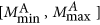. The aftershock rates are shown in Figure 7a, in which the MOL (with p = 1) holds clearly and c is a decreasing function of shear stress. We estimate the c-values by fitting the data with A/(τ + c) using the maximum-likelihood method. As shown in Fig. 7b, the c-value has a negative dependence on the shear stress. We confirm this stress dependence for two volume fractions (ϕ = {0.644, 0.645}) and for two magnitude ranges (MM [3, 4], MA [1, 3] and MM [4, 5], MA [2, 4]). This negative shear-stress dependence of the c-value for aftershocks is consistent with the trend inferred from seismological observations5,34,35.

## Discussion and conclusion

Many systems exhibit crackling noise behaviours that share common properties with earthquake statistics36. However, none of them as yet been able to systematically capture the dependence of both the GR law and the MOL on the level of stress5. Here, we have not only shown that these two fundamental laws of statistical seismology are relevant to avalanches in sheared granular matter, but also they have common dependence on the level of stress.

Concerning the GR law, which is commonly observed in a wide range of materials under different conditions from laboratory experiments to the field, we find scale invariance in the model only for a narrow range of volume fraction (ϕ  0.644). This does not contradict the previous studies, most of which involve different boundary conditions. For example, under constant pressure condition13,15, a system can be self-organised to a critical volume fraction at sufficiently low shear rates. Additionally, in some experiments conducted at constant volume fractions, the GR law hardly approximates avalanche-size distributions if the volume fraction is lower than a critical value16,17. In contrast, the GR law holds for earthquakes irrespective of the details: tectonic setting, depth, rock type and loading rate. We do not have a clear answer about this difference. Nevertheless, the fact that our model can produce self-similar scalings over more than five orders of magnitude at the critical volume fraction is sufficient to differentiate between the GR law and other types of distribution. Hence, we can explore the sensibility of this power-law regime to different conditions, especially to shear stress. In addition, when the GR law is not respected, a characteristic avalanche size emerges, a behaviour that may be compared to the characteristic earthquake distribution37,38.

Concerning aftershocks, we have shown that similar relaxation process may occur in sheared granular media and in the seismogenic crust. In addition, the negative stress dependences of the time delay before the onset of the power-law decay rate is the same as the one observed in real seismicity. This stress dependence is also coincident with the behaviour of some simple models based on a frictional constitutive law39, damage mechanics34, or static fatigue40,41, despite the absence of such physical processes in our granular model. A quantitative result in the present study, that the time constant c in MOL is an exponentially decreasing function of the applied shear stress, should be tested in more realistic and complex systems that are relevant to seismogenic zones as well as various industrial situations.

## Methods

### Simulation model

Our three-dimensional granular system is made of frictionless spheres with diameters of d and 0.7d (the ratio of populations is 1:1). For the sake of simplicity, we assume that the mass m of these particles are the same. We limit ourselves to a rather small-size system (N = 1500) for computational efficiency. Using the radius and the position of particle i, which are denoted by Ri and ri, respectively, the force between particles i and j is written as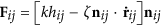. Here rij = ri − rj, nij = rij/|rij| and hij = (Ri + Rj) − |rij| is the overlap length. If Ri + Rj < |rij|, particles i and j are not in contact so that the force vanishes.

Throughout this study, we adopt the units in which d = 1, m = 1 and k = 1. We choose ζ = 2.0, which corresponds to the vanishing coefficient of restitution. A constant shear rateis applied to the system through the Lees-Edwards boundary conditions42. Note that under these boundary conditions the system volume is constant. Thus, the important parameters are the shear rateand the packing fraction ϕ.

To set-up the initial condition, particles are randomly distributed in a simulation box with zero shear stress. When the kinetic energy has relaxed to zero, a constant shear rate is applied. To avoid transient behaviours, we concentrate only on the data for which the the total strain is greater than 100%. Simultaneously, we verify that a steady state of uniform shear rate and uniform volume fraction is achieved. Here, we investigate such uniform steady states only.

### Definition of avalanche

The beginning of an avalanche is taken at time t = t1 at which the total elastic energy E(t) starts decreasing; i.e.,and. Symmetrically, the end of an event is the time t = t2 at which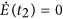and. We also calculate the so-called global shear stressusing the virial43. In analysing the time series, we did not set the noise threshold. Namely, any small increase in energy leads to the termination of an avalanche.

For simplicity, the spatial information of avalanches is discarded. In this case, one might overlook simultaneous avalanches occurring at different places. However, this is unlikely because the present system is small (i.e. the characteristic length is approximately of 9d).

### Definitions of mainshocks and aftershocks

Because aftershocks result from changes of stress induced by a mainshock, we disregard the smaller avalanches for which M < 0 and consider ranges of magnitude for mainshocks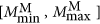and aftershocks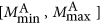. In practice, a magnitude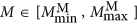event occurring at time tM is not a mainshock if there is at least one avalanche of the same or higher magnitude range in the time interval [tM − ΔT, tM + ΔT]. Thus, we only consider isolated mainshocks and, taking sufficiently large ΔT-values, we also avoid overlapping aftershock sequences that belong to different mainshocks. Then, allavalanches that follow a magnitude MM mainshock within the time interval [tM, tM + ΔT] are regarded as aftershocks. Finally, each aftershock is characterised by its magnitude MA and the elapsed time τ since the mainshock.

In order to reduce artefacts related to event detectability, we disregard larger magnitude range for mainshocks and smaller magnitude range for aftershocks. In addition, the magnitude range of mainshocks are chosen within the intermediate magnitude range in which the GR law holds. Using this strict methodology, we considerably reduce the number of events in our artificial catalogues. Therefore, in all ranges of magnitudes, aftershocks are stacked with respect to the time of their mainshocks to finally end-up with a single aftershock sequence.